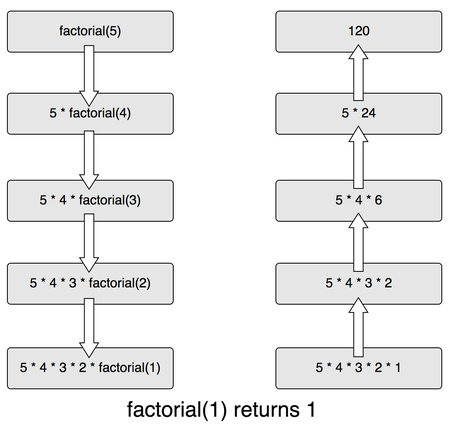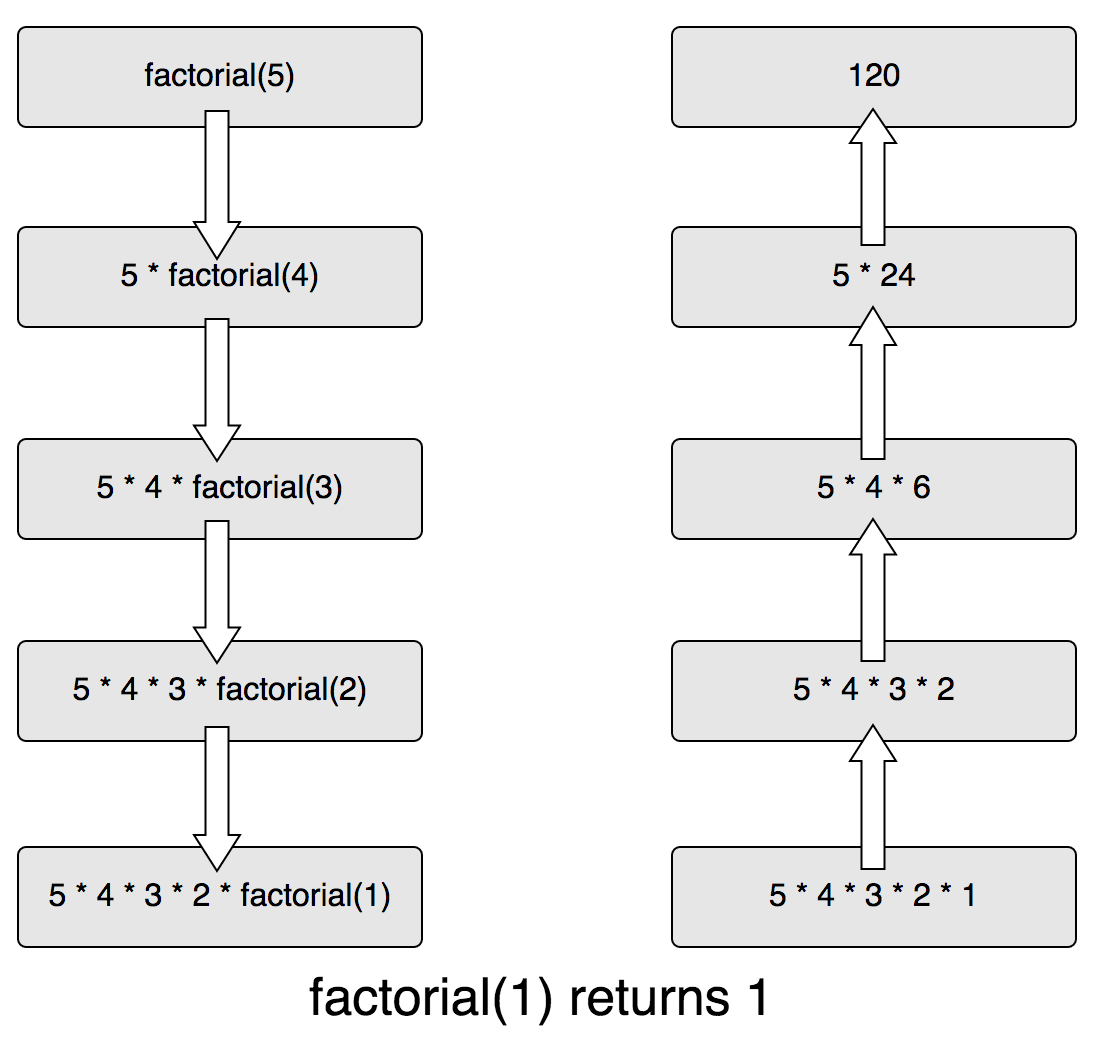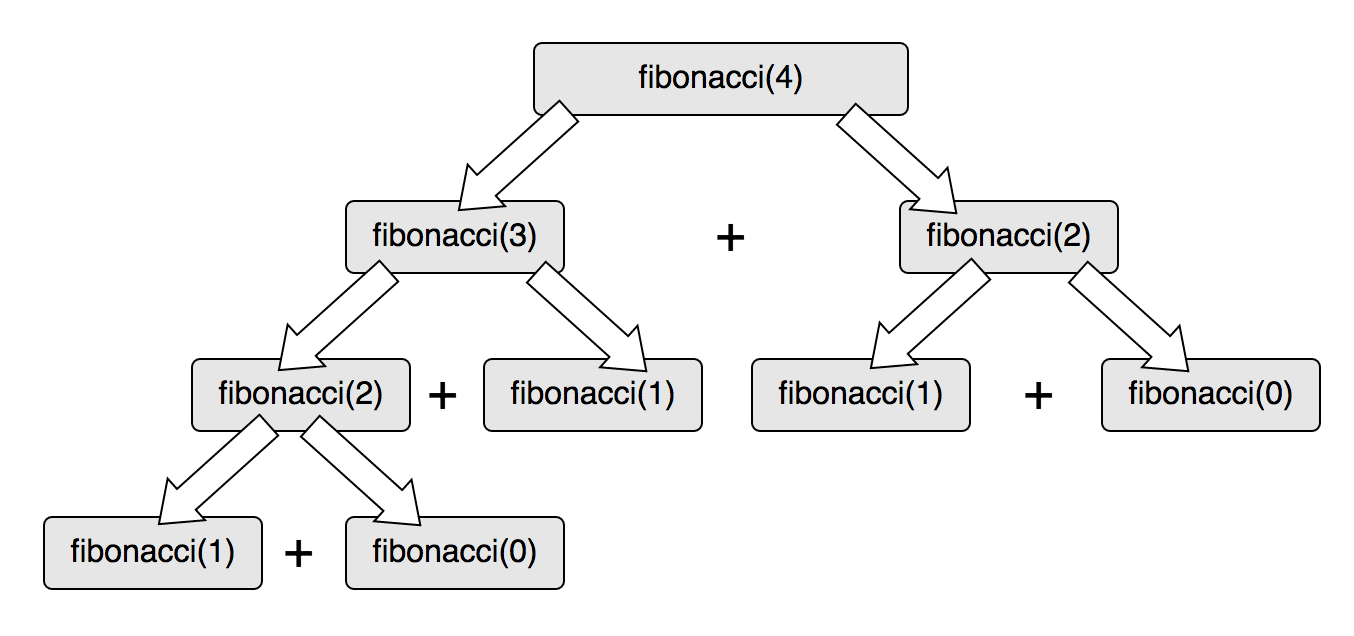## 递归

``````factorial(5) = factorial(4) * 5
factorial(5) = factorial(3) * 4 * 5
factorial(5) = factorial(2) * 3 * 4 * 5
factorial(5) = factorial(1) * 2 * 3 * 4 * 5
factorial(5) = factorial(0) * 1 * 2 * 3 * 4 * 5
factorial(5) = 1 * 1 * 2 * 3 * 4 * 5
``````

## 理解递归``````// N is the Nth fibonacci
function fibonacci(n) {
if (n < 2) {
return 1
} else {
return fibonacci(n - 2) + fibonacci(n - 1)
}
}
``````1. 定义退出条件，也就是自身即结果的原子级定义（也叫“既定结果”）。
2. 定义算法的哪个部分是可递归的

## 递归与调用栈

``````function factorial(n) {
if (n === 0) {
return 1
}

return n * factorial(n - 1)
}
``````

``````factorial(0) // The factorial of 0 is 1 by definition (base case)
factorial(1) // This call depends on factorial(0)
factorial(2) // This call depends on factorial(1)
factorial(3) // This first call depends on factorial(2)
``````

``````function factorial(n) {
console.trace()
if (n === 0) {
return 1
}

return n * factorial(n - 1)
}

factorial(3) // Let's call the factorial function and see what happens
``````

``````Trace
at factorial (repl:2:9)
at repl:1:1 // Ignore everything below this line, it's just implementation details
at realRunInThisContextScript (vm.js:22:35)
at sigintHandlersWrap (vm.js:98:12)
at ContextifyScript.Script.runInThisContext (vm.js:24:12)
at REPLServer.defaultEval (repl.js:313:29)
at bound (domain.js:280:14)
at REPLServer.runBound [as eval] (domain.js:293:12)
at REPLServer.onLine (repl.js:513:10)
at emitOne (events.js:101:20)
``````

``````Trace
at factorial (repl:2:9)
at factorial (repl:7:12)
at repl:1:1 // Ignore everything below this line, it's just implementation details
at realRunInThisContextScript (vm.js:22:35)
at sigintHandlersWrap (vm.js:98:12)
at ContextifyScript.Script.runInThisContext (vm.js:24:12)
at REPLServer.defaultEval (repl.js:313:29)
at bound (domain.js:280:14)
at REPLServer.runBound [as eval] (domain.js:293:12)
at REPLServer.onLine (repl.js:513:10)
``````

``````Trace
at factorial (repl:2:9)
at factorial (repl:7:12)
at factorial (repl:7:12)
at repl:1:1
at realRunInThisContextScript (vm.js:22:35)
at sigintHandlersWrap (vm.js:98:12)
at ContextifyScript.Script.runInThisContext (vm.js:24:12)
at REPLServer.defaultEval (repl.js:313:29)
at bound (domain.js:280:14)
at REPLServer.runBound [as eval] (domain.js:293:12)
``````

``````Trace
at factorial (repl:2:9)
at factorial (repl:7:12)
at factorial (repl:7:12)
at factorial (repl:7:12)
at repl:1:1
at realRunInThisContextScript (vm.js:22:35)
at sigintHandlersWrap (vm.js:98:12)
at ContextifyScript.Script.runInThisContext (vm.js:24:12)
at REPLServer.defaultEval (repl.js:313:29)
at bound (domain.js:280:14)
``````

## 适当的尾调用（PTC）

ES6出来后应该会实现适当的尾调用，但是由于我将在本文后面解释的原因，所有主要的JS引擎目前都没有实现。

``````// If total is not provided we assign 1 to it
function factorial(n, total = 1) {
if (n === 0) {
}

return factorial(n - 1, n * total)
}
``````

1. 在栈顶部压入一个对`factorial`的调用。
2. 因为`4`不是0（既定情况），那么我们知道下一次要计算的值（`3`）和当前累积值（`4 * total`）。
3. 再次调用`factorial`，它会得到完成计算所需的所有数据：要计算的下一个阶乘和累积的总数。至此，不再需要之前的栈帧了，可以把它弹出，只添加新的调用`factorial(3, 4)`
4. 这次调用同样大于0，于是需要计算下一个数的阶乘，同时将累积值（`4`）与当前值（`3`）相乘。
5. 至此（又）不再需要上一次调用了，可以把它弹出，再次调用`factorial`并传入`2``12`。再次更新累积值为`24`，同时计算`1`的阶乘。
6. 前一帧又从栈中被删除，我们又用`1`乘以`24`（总数），并计算`0`的阶乘。
7. 最后，`0`的阶乘返回了累积的总数，也就是`24`（就是4的阶乘）。

``````factorial(4, 1) // 1 is the default value when nothing gets passed
factorial(3, 4) // This call does not need the previous one, it has all the data it needs
factorial(2, 12) // This call does not need the previous one, it has all the data it needs
factorial(1, 24) // This call does not need the previous one, it has all the data it needs
factorial(0, 24) // -> Returns the total (24) and also does not need the previous one
``````

## 在Node中使用适当的尾调用

``````function factorial(n, total = 1) {
console.trace()
if (n === 0) {
}

return factorial(n - 1, n * total)
}

factorial(3)
``````

``````// ...
// These are the last two calls to the `factorial` function
Trace
at factorial (repl:2:9) // Here we have 3 calls stacked
at factorial (repl:7:8)
at factorial (repl:7:8)
at repl:1:1 // Implementation details below this line
at realRunInThisContextScript (vm.js:22:35)
at sigintHandlersWrap (vm.js:98:12)
at ContextifyScript.Script.runInThisContext (vm.js:24:12)
at REPLServer.defaultEval (repl.js:313:29)
at bound (domain.js:280:14)
at REPLServer.runBound [as eval] (domain.js:293:12)
Trace
at factorial (repl:2:9) // The last call added one more frame to our stack
at factorial (repl:7:8)
at factorial (repl:7:8)
at factorial (repl:7:8)
at repl:1:1 // Implementation details below this line
at realRunInThisContextScript (vm.js:22:35)
at sigintHandlersWrap (vm.js:98:12)
at ContextifyScript.Script.runInThisContext (vm.js:24:12)
at REPLServer.defaultEval (repl.js:313:29)
at bound (domain.js:280:14)
``````

``````'use strict'

function factorial(n, total = 1) {
console.trace()
if (n === 0) {
}

return factorial(n - 1, n * total)
}

factorial(3)
``````

```````\$ node --harmony_tailcalls factorial.js`
``````

``````Trace
at factorial (/Users/lucasfcosta/factorial.js:4:13)
at Object.<anonymous> (/Users/lucasfcosta/factorial.js:12:1)
at Module._compile (module.js:571:32)
at Object.Module._extensions..js (module.js:580:10)
at Module.runMain (module.js:605:10)
at run (bootstrap_node.js:420:7)
at startup (bootstrap_node.js:139:9)
Trace
at factorial (/Users/lucasfcosta/factorial.js:4:13)
at Object.<anonymous> (/Users/lucasfcosta/factorial.js:12:1)
at Module._compile (module.js:571:32)
at Object.Module._extensions..js (module.js:580:10)
at Module.runMain (module.js:605:10)
at run (bootstrap_node.js:420:7)
at startup (bootstrap_node.js:139:9)
Trace
at factorial (/Users/lucasfcosta/factorial.js:4:13)
at Object.<anonymous> (/Users/lucasfcosta/factorial.js:12:1)
at Module._compile (module.js:571:32)
at Object.Module._extensions..js (module.js:580:10)
at Module.runMain (module.js:605:10)
at run (bootstrap_node.js:420:7)
at startup (bootstrap_node.js:139:9)
Trace
at factorial (/Users/lucasfcosta/factorial.js:4:13)
at Object.<anonymous> (/Users/lucasfcosta/factorial.js:12:1)
at Module._compile (module.js:571:32)
at Object.Module._extensions..js (module.js:580:10)
at Module.runMain (module.js:605:10)
at run (bootstrap_node.js:420:7)
at startup (bootstrap_node.js:139:9)
``````

## 尾调用优化（TCO）

``````function factorial(n, total = 1) {
if (n === 0) {
}

return factorial(n - 1, n * total)
}
``````

``````function factorial(n, total = 1) {
LABEL:
if (n === 0) {
}
total = n * total
n = n - 1
goto LABEL
}
``````